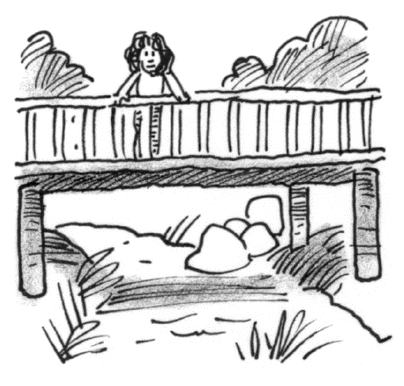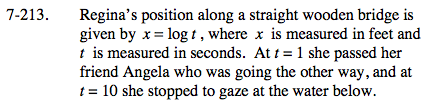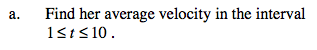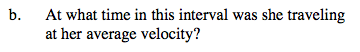### Home > CALC > Chapter 7 > Lesson 7.4.4 > Problem7-213

7-213.
1.Regina's position along a straight wooden bridge is given by x = log t, where x is measured in feet and t is measured in seconds. At t = 1 she passed her friend Angela who was going the other way, and at t = 10 she stopped to gaze at the water below. Homework Help ✎

1. Find her average velocity in the interval 1 ≤ t ≤ 10.

2. At what time in this interval was she traveling at her average velocity?$\text{average velocity}=\frac{\text{displacement}}{\text{time}}$This is an application of the Mean Value Theorem, which guarantees that, on a closed interval, IROC = AROC somewhere if the function is continuous and differentable.